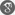##### Cite as:
Toufik Guendouzi, Lamia Bousmaha, Almost Periodic Solutions for Impulsive Fractional Stochastic Evolution Equations, Int. J. Anal. Appl., 6 (1) (2014), 28-43.

#### Abstract

In this paper, we consider the existence of square-mean piecewise almost periodic solutions for impulsive fractional stochastic evolution equations involving Caputo fractional derivative. The main results are obtained by means of the theory of operators semi-group, fractional calculus, fixed point technique and stochastic analysis theory and methods adopted directly from deterministic fractional equations. Some known results are improved and generalized.

#### References

1. J.O. Alzabut, J.J. Nieto, G.T. Stamov, Existence and exponential stability of positive almost periodic solutions for a model of hematopoiesis, Bound Value Probl., 2009 (2009), Article ID 127510.2. S. Abbas, M. Benchohra, G.M. N’Gu´er´ekata, Topics in fractional differential equations. Developments in Mathematics, Springer, New York (2012).3. R.P. Agarwal, B. Andrade, G. Siracusa, On fractional integro-differential equations with state-dependent delay, Comput. Math. Appl., 62 (2011) 1143-1149.4. B. Andrade,J.P.C. Santos, Existence of solutions for a fractional neutral integro-differential equation with unbounded delay, Electron. J. Differ. Equ., 2012 (2012) 1-13.5. P. Bezandry, Existence of almost periodic solutions to some functional integro-differential stochastic evolution equations, Statist. Probab. Lett., 78 (2008) 2844-2849.6. P. Bezandry, T. Diagana, Existence of almost periodic solutions to some stochastic differential equations, Applicable Anal.,86 (7) (2007) 819-827.7. J. Cao, Q. Yang, Z. Huang, On almost periodic mild solutions for stochastic functional differential equations, Nonlinear Anal. RWA., 13 (2012) 275-286.8. Y.K. Chang, R. Ma, Z.H. Zhao, Almost periodic solutions to a stochastic differential equation in Hilbert spaces, Results in Math., 63, 1-2 (2013) 435-449.9. J. Dabas, A. Chauhan, M. Kumar, Existence of the mild solutions for impulsive fractional equations with infinite delay, Int. J. Differ. Equ. 2011 (2011), Article ID 793023.10. G. Da Prato, J. Zabczyk, Stochastic eqnarrays in infinite dimensions, Cambridge University Press, Cambridge (1992).11. A. Debbouche, M.M. El-Borai, Weak almost periodic and optimal mild solutions of fractional evolution equations, Electron. J. Differ. Equ., 46 (2009) 1-8.12. M.M. El-Borai, A. Debbouche, Almost periodic solutions of some nonlinear fractional differential equations, Int. J. Contemp. Math. Sci. 4 (2009), 1373-1387.13. R. Jahanipur, Stochastic functional evolution equations with monotone nonlinearity: existence and stability of the mild solutions, J. Differ. Equations 248 (2010), 1230-1255.14. A.A. Kilbas, H.M. Srivastava, J.J. Trujillo, Theory and Applications of Fractional Differential Equations, Elsevier Science B.V., Amsterdam (2006).15. J. Liu, C. Zhang, Existence and statbility of almost periodic solutions for impulsive differential equations, Adv. Diff. Equa., 2012 (2012), Article ID 34.16. J. Liu, C. Zhang, Existence and statbility of almost periodic solutions to impulsive stochastic differential equations, Cubo, 15, 1 (2013), 77-96.17. J. Luo, Fixed points and exponential stability of mild solutions of stochastic partial differential equations with delays, J. Math. Anal. Appl., 342 (2008) 753-760.18. K.S. Miller, B. Ross, An Introduction to the Fractional Calculus and Differential Equations, John Wiley, New York (1993).19. A. Pazy, Semigroups of linear operators and application to partial differential equations, Springer-Verlag, New York, 1983.20. I. Podlubny, Fractional Differential Equations, Academic Press, San Diego (1999).21. G. Prato, C. Tudor, Periodic and almost periodic solutions for semilinear stochastic evolution equations, Stoch. Anal. Appl., 13 (1995), 13-33.22. A.M. Samoilenko, N.A. Perestyuk, Impulsive differential equations, World Scientific, Singapore, 1995.23. X.B. Shu, Y. Lai, Y. Chen, The existence of mild solutions for impulsive fractional partial differential equations, Nonlinear Anal. TMA 74 (2011), 2003-2011.24. G.T. Stamov, Existence of almost periodic solutions for strong stable impulsive differential equations, IMA J Math Control., 18 (2001), 153-160.25. G.T. Stamov, J.O. Alzabut, Almost periodic solutions for abstract impulsive differential equations, Nonlinear Anal. TMA., 72 (2010), 2457-2464.26. G.T. Stamov, Almost periodic solutions of impulsive differential equations, Springer, Berlin (2012).27. G.T. Stamov, I.M. Stamova, Almost periodic solutions of impulsive fractional differential equations, Dyn. Styt: An Int. J., 29 (2014), 119-132.28. T. Taniguchi, K. Liu, A. Truman, Existence, uniqueness, and asymptotic behavior of mild solutions to stochastic functional differential equations in Hilbert spaces, J. Differ. Equations 181 (2002) 72-91.29. J.R. Wang, M. Feckan M, Y. Zhou, On the new concept of solutions and existence results for impulsive fractional evolution equations, Dyn Partial Differ Equ. 8 (2011) 345-361.30. X. Zhang, X. Huang, Z. Liu, The existence and uniqueness of mild solutions for impulsive fractional equations with nonlocal conditions and infinite delay, Nonlinear Anal. Hybrid Syst. 4 (2010) 775-781.31. Y. Zhou, F. Jiao, Existence of mild solutions for fractional neutral evolution equations, Comput. Math. Appl., 59 (2010), 1063-1077.